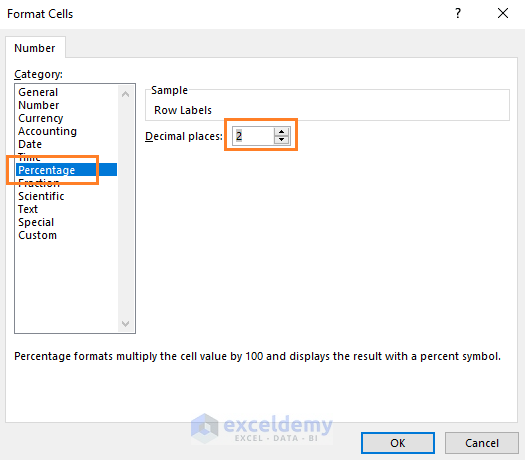Disclosure: This post may contain affiliate links, meaning when you click the links and make a purchase, we receive a commission.

# Excel formula to calculate percentage of grand total (4 Easy Ways)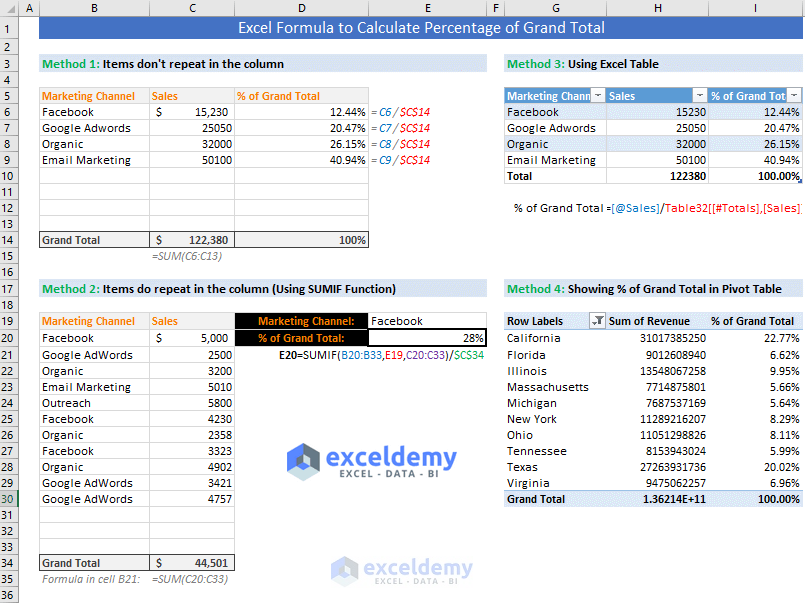In this tutorial, you will learn several methods of using Excel formula to calculate percentage of grand total.

## Basics of Percentage (%)

Percentage (%) is one of the most important mathematical concepts that we use in our daily life. The percentage is a level playing ground. On the basis of this ground, we compare every performance.

Every fraction can be converted to a percentage by multiplying the fraction with 100%. 100% is actually 1.

How?

100%

= 100 x (1/100); [% = 1/100]

= 1

We can multiply any value by 1, so we can multiply any value or fraction by 100% as 100% is actually 1.

An Example

Suppose you bought a stock at \$63 and sold it at \$89. How much did you gain from this stock?

(\$89 – \$63)/\$63 = \$26/\$63 = 0.4127 = 0.4127 x 100% = 41.27%

In our above example, our dollar gain was: \$89 – \$63 = \$26.

When we compare this gain (\$26) with our base value \$63, our gain ratio is: \$26/\$63 = 0.4127

To convert this fraction (or ratio) to a percentage, we have multiplied it by 100%

0.4127 = 0.4127 x 100% = 41.27%

## Percentage in Excel

In Excel, we get percentage using the following formula:

Part/Whole -> Apply Percentage Style on the cell

Note: Keyboard Shortcut to Apply Percent Style on a cell: CTRL + SHIFT + %

Let me explain this concept with an example.

Suppose, you are the manager of several projects. Every project has Sales Target and the year-end Achievement.

To find out the Percentage (%) of Achievement, follow these steps:

• In the cell D2, input this formula: =C2/B2 and press Enter key on your keyboard.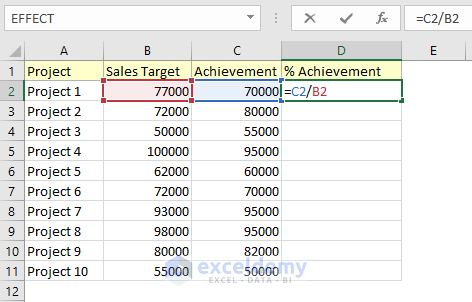• Then copy the formula for other cells (D3:D11) in the column.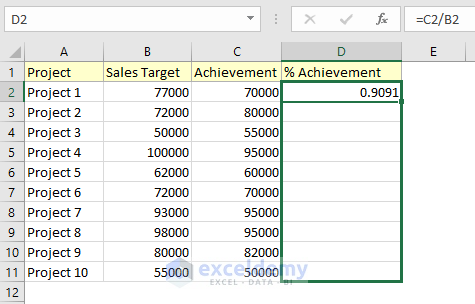• Now select the cell range (D2:D11) and apply Percent Style format (keyboard shortcut: CTRL + SHIFT + %) from the Number group of commands in the Home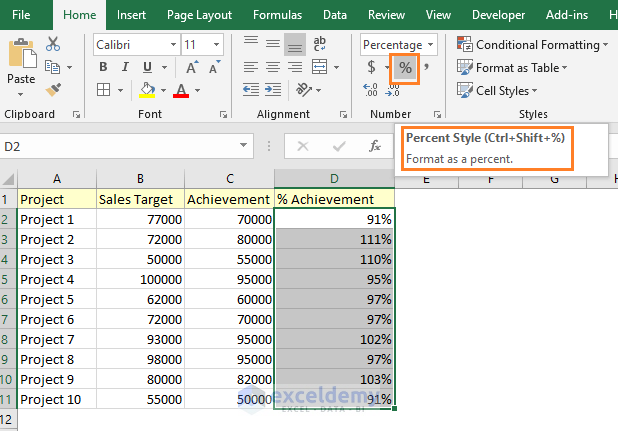• If necessary, you can increase or decrease the decimal points using two commands in the same group (Home -> Number): Increase Decimal and Decrease Decimal.
• You’re done!

So, this is how the percentage works in the Excel environment.

## How to calculate the percentage of grand total in Excel

In many cases, you might need to calculate the percentage of Grand Total. In Excel, you can do it with the blink of an eye.

Calculating the percentage of Grand Total can be two types:

• First type: Items will not repeat in the column. The Grand Total will be at the end of the column and in a fixed cell. We will calculate the percentage based on that cell.
• Second type: In the Grand Total column, the items can repeat. But Grand Total will be at the end of the column and in a fixed cell.

### 1) First type: Items don’t repeat in the column

To explain this scenario, we have taken the example of a digital company that generates sales in more than one way.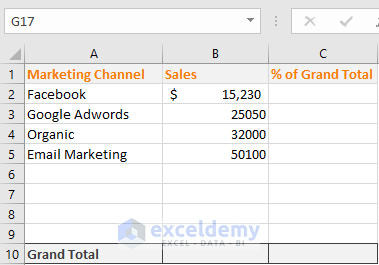Follow these steps to calculate the percentage of Grand Total:

• At the end of the year, the company has calculated their total sales (Grand Total) from different channels. In the cell B10, we have used this formula: =SUM(B2:B9) to calculate the total sales (Grand Total).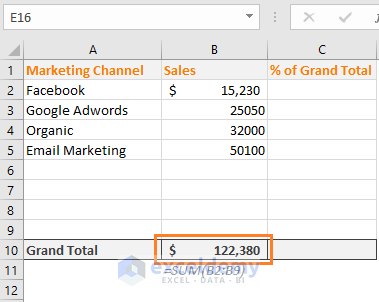• The performance of any channel can be evaluated in this way: (The sales generated by a channel / Total Sales generated by all the channels) x 100%. That is, you’re seeing in the following image.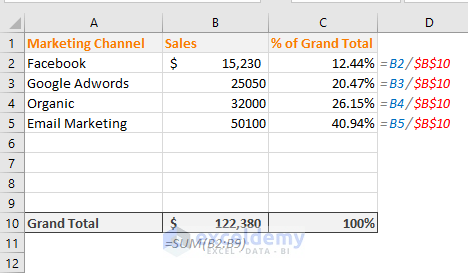You see from the above image that the Grand Total cell (B10) is an absolute reference in the formulas. This is because, when we will copy the formula for other cells in the column, the cell reference B10 will be unchanged.

I have kept some rows (row 6, 7, 8, and 9) blank. This is because: later when you would use more channels to generate sales, you would be able to add them in those blank rows.

### 2) Second type: Items can repeat in the column

In this example, I will show how to calculate the percentage of Grand Total when the item can repeat in the column.

See the data below. The marketing channels are repeating in the column. For example, Facebook has repeated 5 times, Google AdWords has repeated 4 times and so on. At the end of the column, you’re seeing the total sales from all the channels.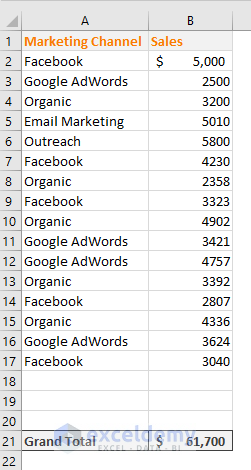Follow these steps to get the percentage of Grand Total from the above data:

Step 1: Calculate Grand Total

In the cell B21, calculate the total sales using this formula: =SUM(B2:B20).

Step 2: Find out the unique records in the column

Now let’s find out how many unique records are in the column.

• Select the range A1:A17 -> Open the Data ribbon -> Click on the Advanced command in the Sort & Filter group of commands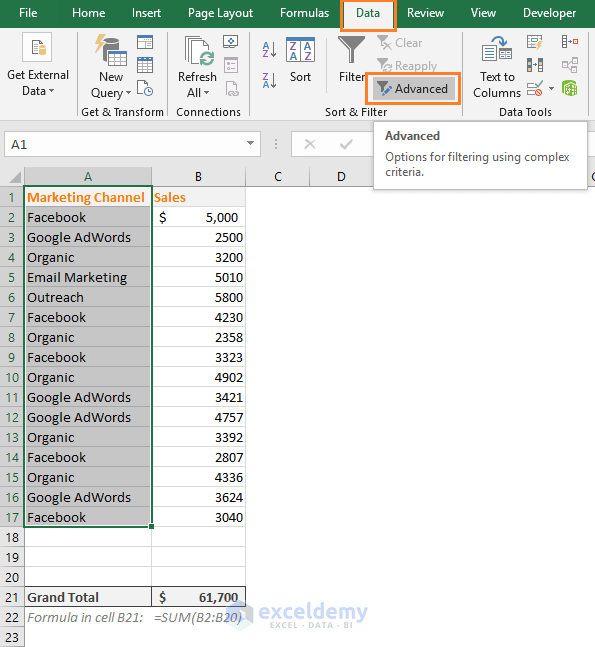• Advanced Filter dialog box will appear. Choose Copy to another location option -> In the List range field, range \$A\$1:\$A17 is already set -> In the Copy to field, input a cell where you want to place the unique records (I choose G1) -> Check mark Unique records only option -> Finally click on the OK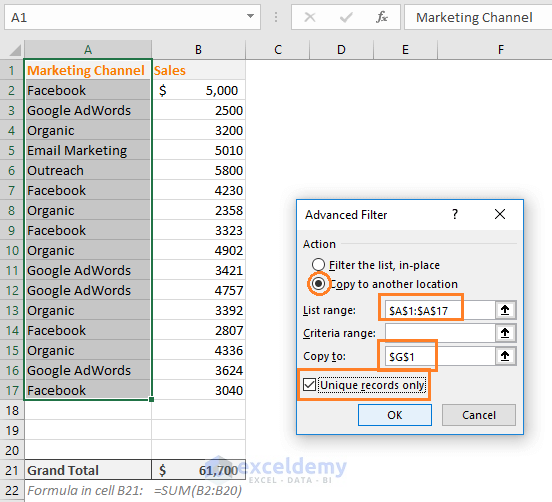• This is what you get.Step 3: Make a named range with the unique records

• Now select the unique records (created in step 2) -> Open the Formulas ribbon -> click on the Define Name command in the Defined Names group of commands.• New Name dialog box will appear. Keep the options as it is. Just click on the OK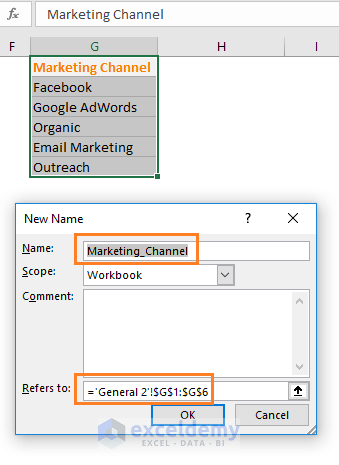Step 4: Hide the unique records column

The data of column G (where unique records are placed) are intermediary. So, we hide the G column. This action is not required but it is a good practice.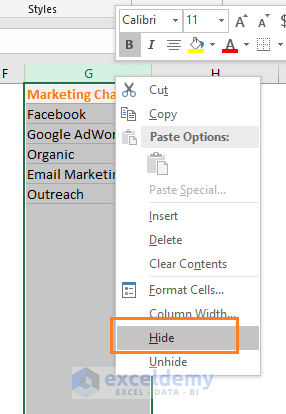Step 5: Make a drop-down list with the unique records

• Select the cell E1 -> open the Data ribbon -> click on the Data Validation command in the Data Tools group of commands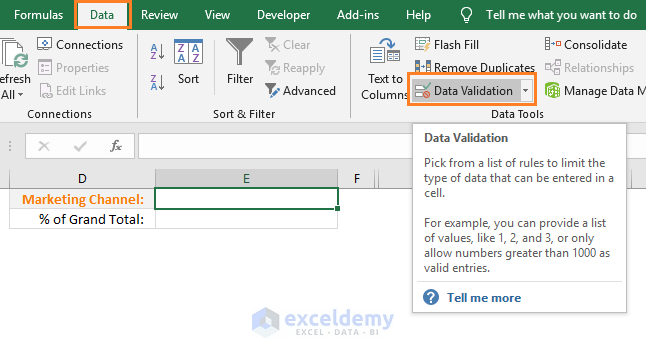• Data Validation dialog box appears. From the Allow drop-down, select the List option -> In the Source field, input this formula: =Marketing_Channel -> Finally, click on the OK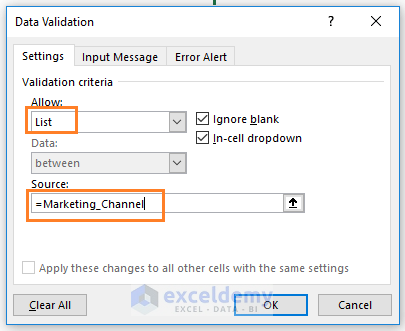• In the cell E1, you see a drop-down has been created.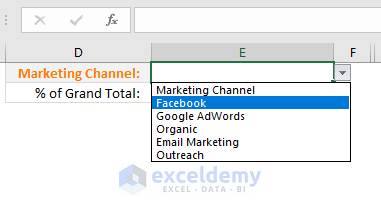Step 6: Calculate the percentage of Grand Total

In the cell E2, input this formula: =SUMIF(A2:A20,E1,B2:B20)/\$B\$21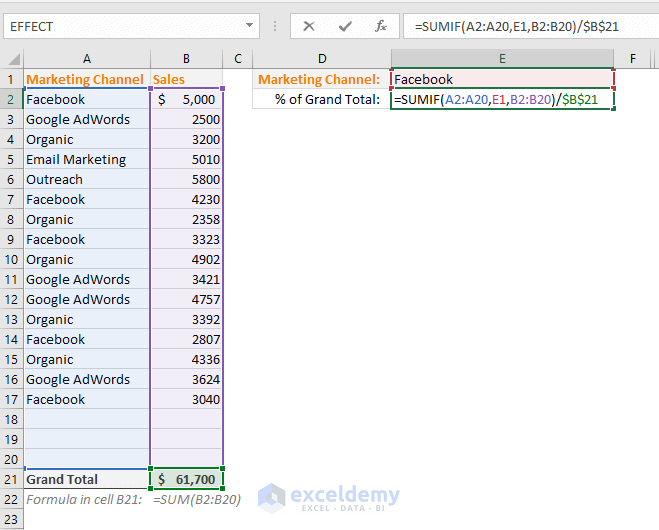In the above formula, we have calculated the total sales using the Facebook channel and divided it by the Grand Total:

=SUMIF (range, criteria, sum_range) / Grand Total

To understand this formula, you have to understand how SUMIF function works.

SUMIF Function adds the cells specified by a given condition or criteria.

Syntax of SUMIF function: SUMIF(range, criteria, [sum_range])

In our example, range is A2:A20, the criterion is the cell reference E1 and sum range is B2:B20.

Total sales using the Facebook channel is: \$18400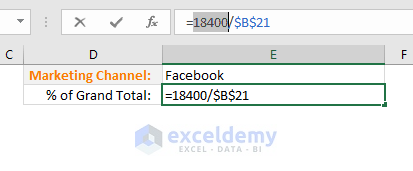How did I find that?

I have just selected the SUMIF function part in the formula and then have pressed the F9 key in the keyboard.

Internally, Excel divides the 18400 value by the total sales 61700. As the cell is formatted with Percent Style, we see the percentage value in the cell E2.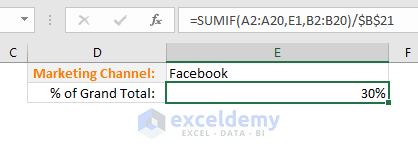This happens when you change the Marketing Channel.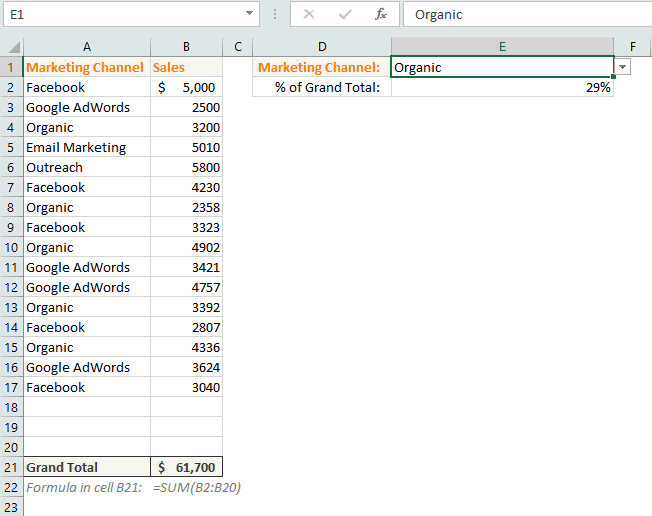## 3) Calculating the percentage of Grand Total using Excel Table

You can also use an Excel table to calculate the percentage of Grand Total.

Step 1: Converting the range into a table

• Select a cell in the range -> In the Insert ribbon, click on the Table command from the Tables group of commands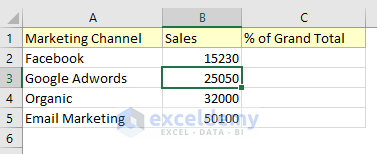• Create Table dialog box will appear -> Keep the options as it is and click on the OK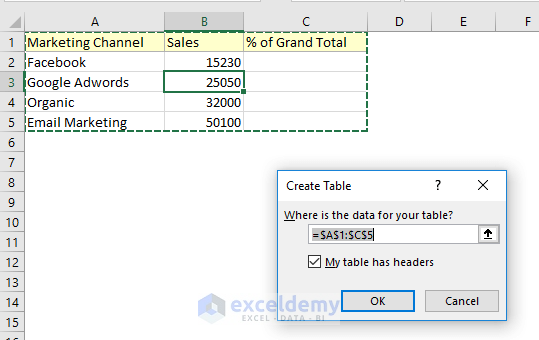• Your table is created. Selecting a cell within the table, choose the Total Row option from the Design Design tab appears only when a table is selected.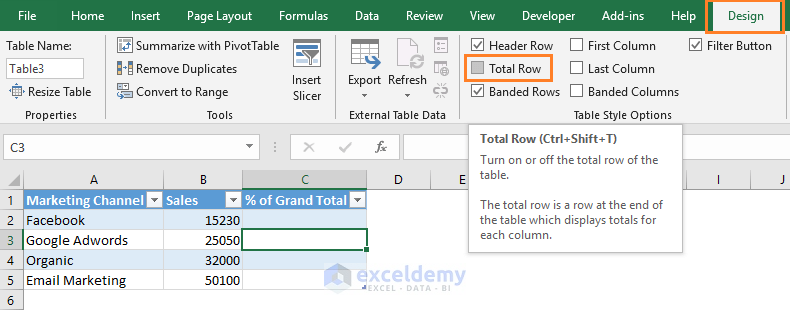• Now show the total values under the column.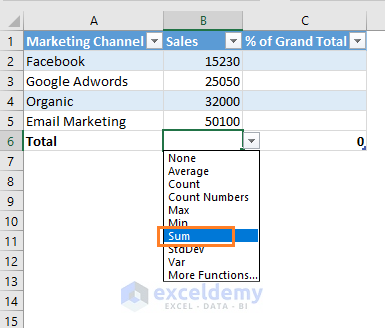• This is what we get.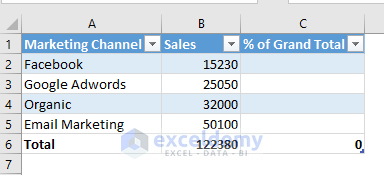Step 2: Calculating the percentage of grand total

Select the first cell (C2) of the % of Grand Total column -> Input an equal sign (=) -> Select the first cell (B2) of the Sales column -> Input the division symbol (/) -> then select the Total cell (B6) of the Sales column -> Finally, click on the OK button.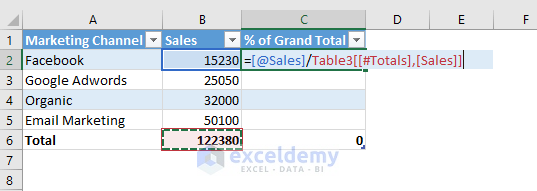This is what we get after applying the Percent Style in the column % of Grand Total.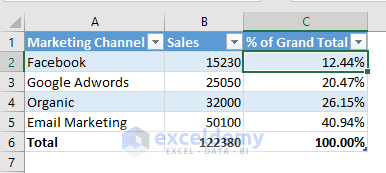Important: Benefits of using a Table instead of using a range

Adding a new row is easy in a table. Select the last cell in the table (excluding the total row) and press Tab key on your keyboard. A new row will be created with the formula.

## 4) How to calculate the percentage of grand total in Excel Pivot Table

Here is some dummy data.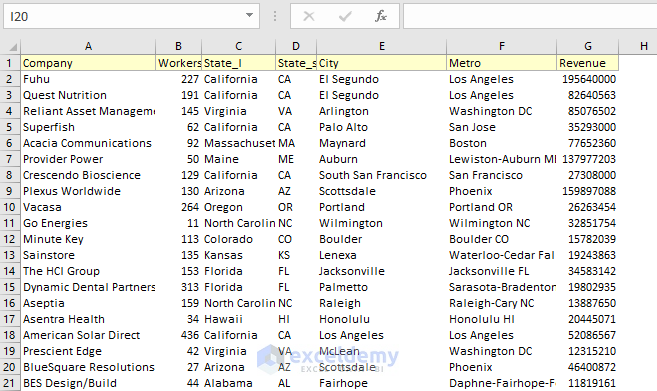Let me show you how to calculate the percentage of grand total in Excel pivot table. Follow these steps:

Step 1: Creating the pivot table

• Select a cell within the range -> Open the Insert tab -> and choose the PivotTable command from the Tables group of commands.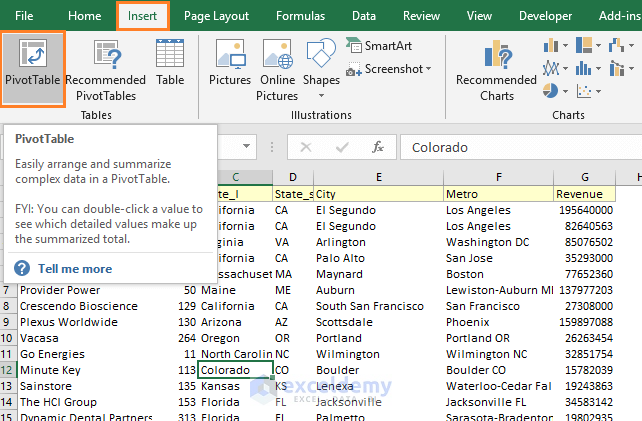• Create PivotTable dialog box appears. Keep the options as it is. If you want to know more about creating a pivot table, read this guide. Click on the OK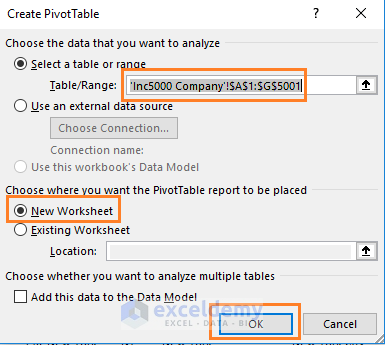• I arrange the Pivot Table fields in the following way (image below). Notice that I have placed the Revenue field two times in the Values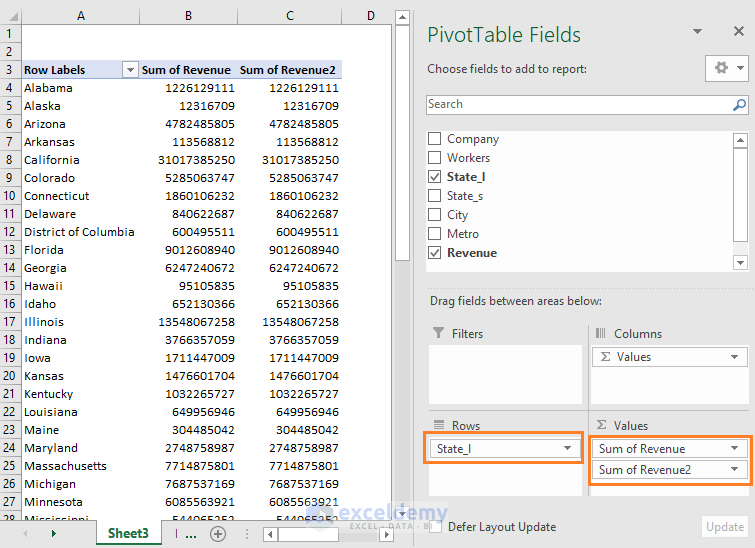Step 2: Calculating percentage of grand total

• Click on the Sum of Revenue2 field in the Values A drop-down will appear. Choose the Value Field Settings… option from the menu.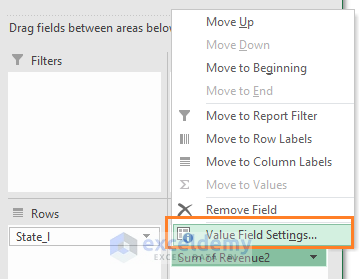• Value Field Settings dialog box will appear. Change the Custom Name field as % of Grand Total -> Choose the Show Values As tab -> Select the option % of Grand Total from the Show values as drop down -> Finally, click on the OK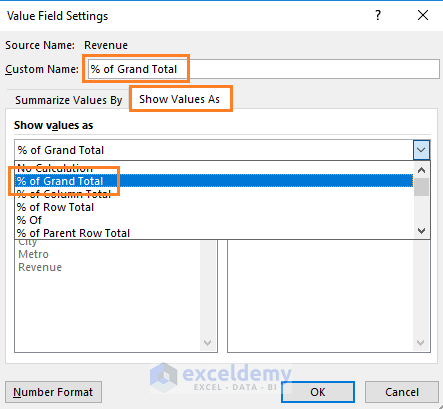• You see % of Grand Total is created in the pivot table.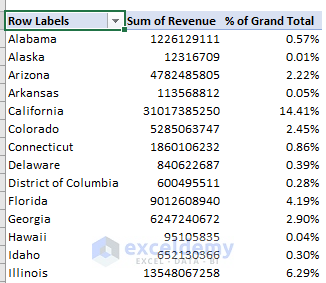Note: In Excel 2016, % of Grand Total column shows the values in percentages. If you’re using a version where it does not show the percentages automatically, here is the solution.

Open the Value Field Settings dialog box of the % of Grand Total field -> Click on the Number Format command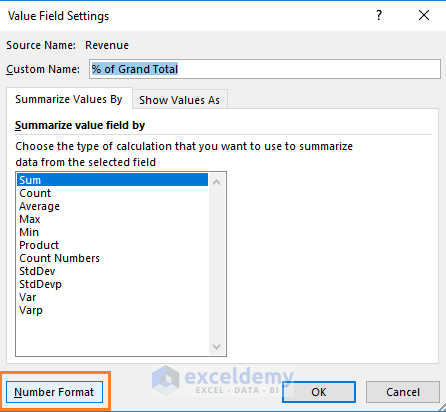Format Cells dialog box will appear. Open the Percentage window and make changes as per your need.